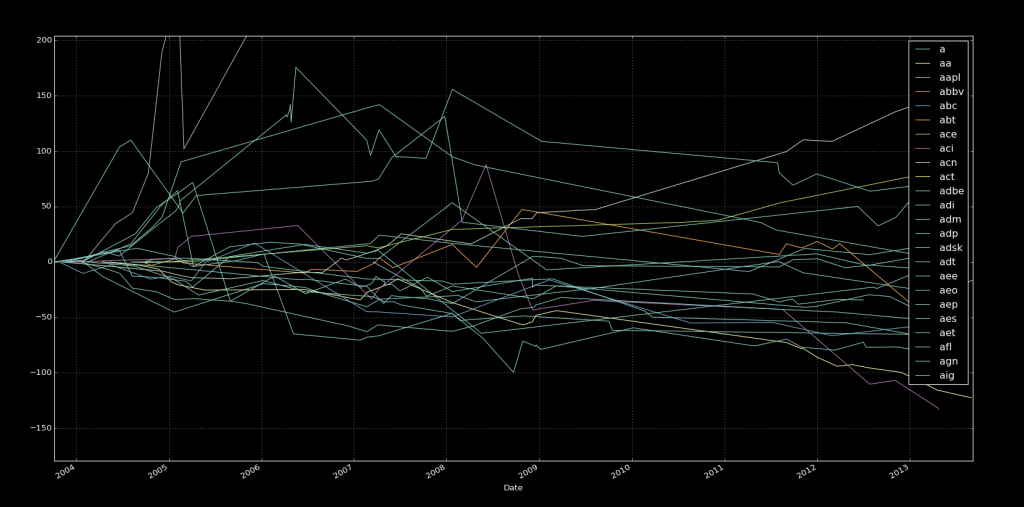## Labeling data part 2

With machine learning, everything tends to boil down to features and labels. We have labels, like, in our case, under-performer, and out-performer. With those labels, we have "features" that are the specific values like Debt/Equity ratio that correspond to that label.

With that, we're looking to now label our data. To do that, we're going to compare the stock's percentage change to the S&P 500's percentage change. If the stock's percent change is less than the S&P 500, then the stock is and under-performing stock. If the percentage change is more, than the label is out-perform.

To do this, we need the calculate percentage change and compare them. Let's cover that:

```import pandas as pd
import os
import time
from datetime import datetime

path = "X:/Backups/intraQuarter"

def Key_Stats(gather="Total Debt/Equity (mrq)"):
statspath = path+'/_KeyStats'
stock_list = [x for x in os.walk(statspath)]
df = pd.DataFrame(columns = ['Date',
'Unix',
'Ticker',
'DE Ratio',
'Price',
'stock_p_change',
'SP500',
'sp500_p_change'])
```

Notice the new changes to our Data Frame.

Next:

```    sp500_df = pd.DataFrame.from_csv("YAHOO-INDEX_GSPC.csv")

ticker_list = []

for each_dir in stock_list[1:25]:
each_file = os.listdir(each_dir)
ticker = each_dir.split("\\")
ticker_list.append(ticker)

starting_stock_value = False
starting_sp500_value = False
```

Notice the starting_stock_value and the matching sp500 version. The reason for this is that, as we go, we want to calculate % change. That said, we need to start over with the % change each time the stock itself changes. To handle for this, we set these values.

Next:

```        if len(each_file) > 0:
for file in each_file:
date_stamp = datetime.strptime(file, '%Y%m%d%H%M%S.html')
unix_time = time.mktime(date_stamp.timetuple())
full_file_path = each_dir+'/'+file
try:
value = float(source.split(gather+':</td><td class="yfnc_tabledata1">').split('</td>'))

try:
sp500_date = datetime.fromtimestamp(unix_time).strftime('%Y-%m-%d')
row = sp500_df[(sp500_df.index == sp500_date)]
except:
sp500_date = datetime.fromtimestamp(unix_time-259200).strftime('%Y-%m-%d')
row = sp500_df[(sp500_df.index == sp500_date)]

stock_price = float(source.split('</small><big><b>').split('</b></big>'))
#print("stock_price:",stock_price,"ticker:", ticker)

if not starting_stock_value:
starting_stock_value = stock_price
if not starting_sp500_value:
starting_sp500_value = sp500_value
```

So now we set the starting value if we don't have one. From here, we then just need to calculate % change (new-old)/old * 100:

```                    stock_p_change = ((stock_price - starting_stock_value) / starting_stock_value) * 100
sp500_p_change = ((sp500_value - starting_sp500_value) / starting_sp500_value) * 100
```

Now we just round off the script with the previously covered code:

```
df = df.append({'Date':date_stamp,
'Unix':unix_time,
'Ticker':ticker,
'DE Ratio':value,
'Price':stock_price,
'stock_p_change':stock_p_change,
'SP500':sp500_value,
'sp500_p_change':sp500_p_change}, ignore_index = True)
except Exception as e:
pass
#print(str(e))

save = gather.replace(' ','').replace(')','').replace('(','').replace('/','')+('.csv')
print(save)
df.to_csv(save)

Key_Stats()
```

The next tutorial:• Intro to Machine Learning with Scikit Learn and Python

• Simple Support Vector Machine (SVM) example with character recognition

• Our Method and where we will be getting our Data

• Parsing data

• More Parsing

• Structuring data with Pandas

• Getting more data and meshing data sets

• Labeling of data part 1

• Labeling data part 2
• Finally finishing up the labeling

• Linear SVC Machine learning SVM example with Python

• Getting more features from our data

• Linear SVC machine learning and testing our data

• Scaling, Normalizing, and machine learning with many features

• Shuffling our data to solve a learning issue

• Using Quandl for more data

• Improving our Analysis with a more accurate measure of performance in relation to fundamentals

• Learning and Testing our Machine learning algorithm

• More testing, this time including N/A data

• Back-testing the strategy

• Pulling current data from Yahoo

• Building our New Data-set

• Searching for investment suggestions

• Raising investment requirement standards

• Testing raised standards

• Streamlining the changing of standards